# Prime divisors

Find 2/3 of the sum's ratio and the product of all prime divisors of the number 120.

x =  0.2222

### Step-by-step explanation: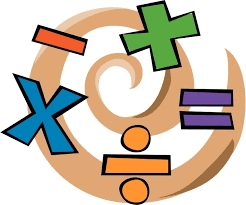Did you find an error or inaccuracy? Feel free to write us. Thank you!Tips to related online calculators
Need help to calculate sum, simplify or multiply fractions? Try our fraction calculator.
Do you want to perform natural numbers division - find the quotient and remainder?

## Related math problems and questions:

• Divisors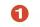Find all divisors of number 493. How many are them?
• Reminder and quotientThere are given numbers A = 135, B = 315. Find the smallest natural number R greater than 1 so that the proportions R:A, R:B are with the remainder 1.
• No. of divisorsHow many different divisors have number 13 4 * 2 4?
• A number 5A number is divisible by 24, 25, and 120 if it is increased by 20. Find the number.
• 7 digit numberIf 3c54d10 is divisible by 330, what is the sum of c and d?
• Quotient 3If the quotient of 8/13 and 2 is subtracted from the product of 1 3/4 and 8/21, what is the difference?
• Fractions and mixed numerals(a) Convert the following mixed numbers to improper fractions. i. 3 5/8 ii. 7 7/6 (b) Convert the following improper fraction to mixed number. i. 13/4 ii. 78/5 (c) Simplify these fractions to their lowest terms. i. 36/42 ii. 27/45 2. evaluate following ex
• Twenty-fiveHow many are three-digit natural numbers divisible by 25?
• Unknown numberIf I reduced the sum of the numbers 70 and the unknown number three times, I would get 100. what is the unknown number?
• Divisibility by 12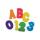Replace the letters A and B by digits so that the resulting number x is divisible by twelve /find all options/. x = 2A3B How many are the overall solutions?
• What is 9What is the value of x in the proportion 2 and one-fourth over x = 1 and one-half over 3 and three-fifths? 2 and two-fifths 5 and two-fifths 8 and 1 over 10 12 and 3 over 20
• Ratio three numbers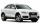Three numbers SUV are in the ratio 1:2:3. Their sum is 24. Find this numbers and write their add and sum.
• DivisorsThe sum of all divisors unknown odd number is 2112. Determine sum of all divisors of number which is twice of unknown numbers.
• NumbersDetermine the number of all positive integers less than 4183444 if each is divisible by 29, 7, 17. What is its sum?
• The product 2The product of two functions is 10. If one of them is 2 1/3, find the other one.
• ErikThree friends Peter, Erik and Milan shared 420 euros in a ratio of 1: 2: 3. How many euros did Erik get?
• Decomposition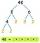Make decomposition using prime numbers of number 155. Result write as prime factors (all, even multiple)